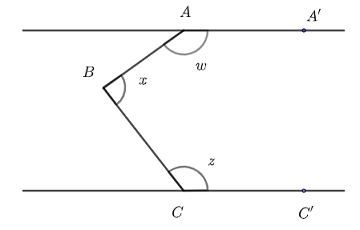# Angles in Parallel Lines and Transversals Problems

Problems related to angles made by parallel lines and transversal are presented below along with their detailed solutions.

## Problem 1

In the figure below, AA' is parallel to CC'. The size w of angle A'AB is equal to 135 degrees and the size z of angle C'CB is equal to 147 degrees. Find angle ABC.Solution to Problem 1:

• Draw BB' parallel to AA' and CC'as shown in the figure below.• Note that angle ABC is given by
angle ABC = angle ABB' + angle CBB'
• Angle w' and angle ABB' are alternate interior angles and their sizes are equal.
angle ABB' = angle w'
• Angle z' and angle CBB' are alternate interior angles and their sizes are equal.
angle CBB' = angle z'
• Angles w and w' are supplementary which gives
w' = 180 - w = 180 - 135 = 45 0
• Angles z and z' are also supplementary which gives
z' = 180 - z = 180 - 147 = 33 0
• We now substitute angle ABB' by w' and angle CBB' by z' in angle ABC = angle ABB' + angle CBB' found above.
angle ABC = w' + z' = 45 + 33 = 78 0

## Problem 2

In the figure below lines A'A" and C'C" are parallel. AB is the bisector of angle CAA" and BC is the bisector of angle ACC". Show that the size of angle ABC is equal to 90 degrees.Solution to Problem 2:

• Angles A'AC and angle ACC" are alternate interior angles and their sizes are equal.
angle A'AC = angle ACC"
• Angles A'AC and angle ACC" are alternate interior angles and their sizes are equal.
angle A'AC = angle ACC"
• Angles A'AC and angle A"AC are supplementary so that
angle A"AC = 180 - angle A'AC = 180 - angle ACC"
• Rearrange the above to obtain
angle A"AC + angle ACC" = 180 0
• Because AB and CB are bisectors(they divide the angle into two equal angles), angle ABC in triangle ABC is given by
angle ABC = 180 - (angle A"AC + angle ACC") / 2
= 180 - 180 / 2 = 90 0

## Problem 3

In the figure below lines BC and DD' are parallel. The size of angle x is equal to 127 degrees and the size of angle y is equal to 115 degrees. Find all interior angles of triangle ADD'.Solution to Problem 3:

• Angle x and angle ABC are supplementary hence
angle ABC = 180 - x = 180 - 127 = 53 0
• Angle y and angle ACB are supplementary hence
angle ACB = 180 - y = 180 - 115 = 65 0
• Angle ADD' and angle ABC are corresponding angles and their sizes are equal
angle ADD' = angle ABC = 53 0
• Angle AD"D and angle ACB are corresponding angles and their sizes are equal
angle AD'D = angle ACB = 65 0
• Angle DAD' is given by